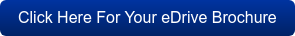What is a Power Analyzer? The Working Principle Explained What is a Power Analyzer? The Working Principle Explained | HBM

# What is a Power Analyzer?

A power analyzer is used to measure the flow of power (w) in an electrical system. This refers to the rate of electrical transferral between a power source and a sink, hence the alternative expression of power as energy per second (J/s). Measuring power flow is a critical yet rudimentary process that can be carried out with consummate ease using a standard power analyzer. More advanced systems acquire electrical signals and carry out integrated calculations for additional, complex analysis.

## The Working Principles of Power Analyzers

Power analyzers can be used to measure the flow of energy in either alternating current (AC) or direct current (DC) systems – with distinct considerations for measuring AC circuits.

The determination of an electrical signal’s True RMS time period underlines each of the subsequent calculations performed by the measuring instrument. This is complicated by AC measurements, where root mean square is typically expressed as an equivalent DC value. To accurately determine the True RMS of an AC waveform, an average must be calculated across the cycle of the AC frequency. This is defined as the fundamental frequency of the circuit. Power analyzers can digitally detect frequency cycles to provide reliable RMS periods during power conversion.

A power analyzer must also detect the voltage and current of the system. Typical systems directly acquire individual voltages using voltage dividers, while a transformer is usually required to measure the current. This may comprise a coil that measures the electrical field of a wire carrying a current, or a flux gate current transducer.

Once the power analyzer has determined each of these values, calculating power is a matter of simple mathematics.

As mentioned, innovative power analyzers provide more than just power measurements. They are often required to measure various values of mechanical energy such as torque and speed, which are critical factors for test bench and manufacturing applications. This provides reliable data for comprehensive investigations into the performance and efficiency of electromechanical systems. Some of the additional calculations and analytical methods performed using advanced power analyzers include:

• Efficiency mapping;
• Fast Fourier Transform (FFT) and harmonic analysis;
• Fundamental power and root mean square (RMS) values;
• Polar diagrams and symmetrical components;
• Space vectors and DQ-currents.

## Power Analyzers from HBM

HBM is one of the world’s leading manufacturers and suppliers of electrical and mechanical measuring instrumentation for industrial facilities. We provide a broad range of power analyzers equipped with our class-leading torque transducers, for ongoing measurement of electric drives of up to 51 power with as many as 6 torque channels.